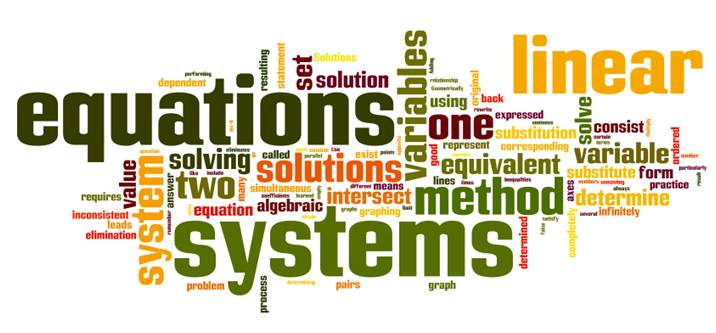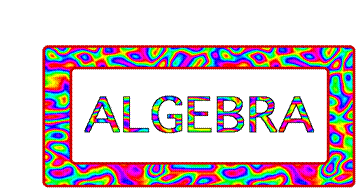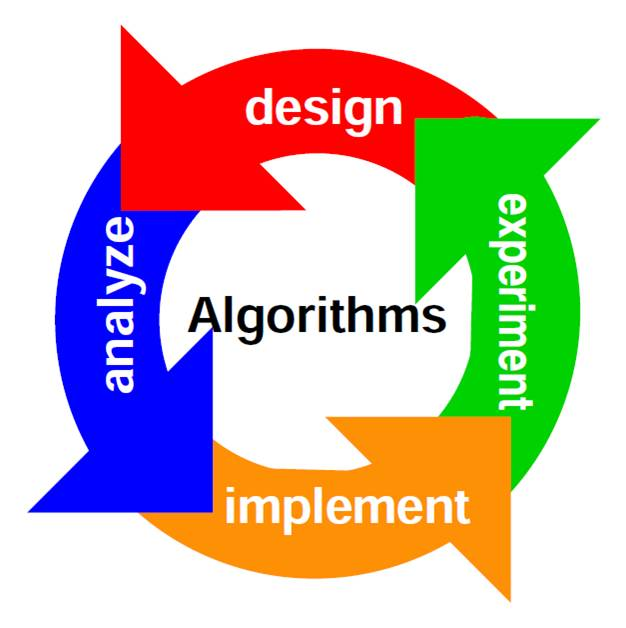• FIND A DEGREE
Degreequery.com is an advertising-supported site. Clicking in this box will show you programs related to your search from schools that compensate us. This compensation does not influence our school rankings, resource guides, or other information published on this site.##### IMAGE SOURCE

“Mathematics is the gate and key to the sciences.”
– Roger Bacon

Mr. Bacon preceded the advent of the computer age by almost 400 years. But his statement could refer to computer science. A degree in computer science typically entails calculus courses, some form of discrete mathematics, and (most likely) a course in real analysis. The type of math varies depending on your specialization within the realm of computer science. For example, discrete mathematics and number theory are extremely important in cryptography studies and network security.

## What is Discrete Math?

The mathematics of modern computer science consists of discrete math, in particular, combinatorics (the theory of ways things combine), sequences, symbolic logic, and graph theory. This means that in order to learn the fundamental algorithms used by computer programmers, students will need a solid background in these subjects. Indeed, at most universities, an undergraduate-level course in discrete mathematics is a required part of pursuing a computer science degree. The study of topics in discrete mathematics usually includes the study of algorithms, their implementations, and efficiencies.

Examples of Mathematics at different degree levels:

##Associate Degree

1. An Associate of Science or Applied Science in Cyber and Network Security degree has one math course. Of the total 63 credits, there are three credit hours of College Algebra. This course examines algebraic applications and problem-solving skills to include the ability to formulate, use, and interpret mathematical models. Topics include properties of the real numbers, graphing of equations and inequalities, the algebra of rational expressions, and properties of exponentials and logarithms.
2. An Associate of Applied Science in Cyber Security has the requirement of a college algebra course. The emphasis is on the study of functions and their graphs, inequalities, and linear, quadratic, rational, polynomial, exponential, and logarithmic functions. An intermediate math course is necessary for students who did not complete two years of algebra and one year of geometry in high school.

##Bachelor’s Degree

At this level, you can expect more mathematics.

1. Bachelor of Science in Cybersecurity and Information Assurance has math courses in the General Education part of the curriculum. One is intermediate algebra that provides an introduction of algebraic concepts through the study of the real number system, linear equations, graphing, and exponents. In addition, there is a course in college algebra and probability and statistics. The latter involves statistical reasoning, and analyzing data.
2. An online Bachelor of Science in Cyber Security has several math courses listed under the General Education requirements. These include an introduction to algebra, analytics college algebra, data-driven statistics, and applications of discrete mathematics. These are 4.5 credit hours each. The core courses don’t require any mathematics.

##Master’s Degree

The good news is that once you’ve attained this level, there is typically less math. Of course, you require a bachelor’s degree to apply to a graduate program. However, the coursework for a Master of Science in Cyber Security will concentrate on courses directly related to this specialty. Examples are courses in data protection, network security, digital forensics, cryptography, e-commerce security, and more.

There are graduate programs that might have a core course in Design and Analysis of Algorithms. An Algorithm is a sequence of steps to solve a problem. Design and Analysis of Algorithms are very important for designing specific algorithms to solve different types of problems in the branch of computer science and information technology.

You may encounter algorithms at the undergraduate level as well. An algorithm is a well-defined procedure that allows a computer to solve a problem. A very simple example of an algorithm would be to find the largest number in an unsorted list of numbers. If you had a list of five different numbers, you would have this figured out in no time, no computer needed. What about a million different numbers? This would require a computer and a computer needs an algorithm to make this determination.

## Prerequisites

You must be a high school graduate or have completed a GED and have earned three and a half units of mathematics (selected from any combination of algebra, geometry, and trigonometry or higher math course) to satisfy the initial admission requirements to be admitted to the College of Information Sciences and Technology. These are the requirements for applicants at one U.S. university.

If you are interested in working in this field at the FBI, then you should have a strong math background. The FBI has a nationwide pilot program, as of 2017, in which agents are working to encourage the study and development of science, technology, engineering, and mathematics courses in high school. The idea is to get students engaged in exercises and other activities that can help prepare them for a career in cyber security.